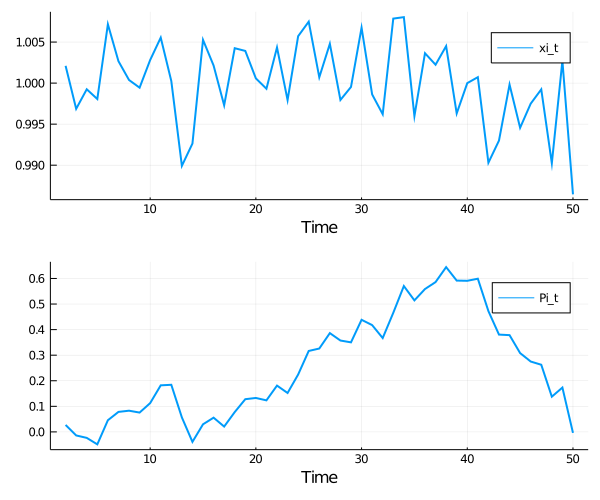# Optimal Taxation in an LQ Economy¶

## Overview¶

In this lecture we study optimal fiscal policy in a linear quadratic setting.

We slightly modify a well-known model of Robert Lucas and Nancy Stokey [LS83] so that convenient formulas for solving linear-quadratic models can be applied to simplify the calculations.

The economy consists of a representative household and a benevolent government.

The government finances an exogenous stream of government purchases with state-contingent loans and a linear tax on labor income.

A linear tax is sometimes called a flat-rate tax.

The household maximizes utility by choosing paths for consumption and labor, taking prices and the government’s tax rate and borrowing plans as given.

Maximum attainable utility for the household depends on the government’s tax and borrowing plans.

The Ramsey problem [Ram27] is to choose tax and borrowing plans that maximize the household’s welfare, taking the household’s optimizing behavior as given.

There is a large number of competitive equilibria indexed by different government fiscal policies.

The Ramsey planner chooses the best competitive equilibrium.

We want to study the dynamics of tax rates, tax revenues, government debt under a Ramsey plan.

Because the Lucas and Stokey model features state-contingent government debt, the government debt dynamics differ substantially from those in a model of Robert Barro [Bar79].

The treatment given here closely follows this manuscript, prepared by Thomas J. Sargent and Francois R. Velde.

We cover only the key features of the problem in this lecture, leaving you to refer to that source for additional results and intuition.

### Model Features¶

• Linear quadratic (LQ) model
• Representative household
• Stochastic dynamic programming over an infinite horizon
• Distortionary taxation

### Setup¶

In :
using InstantiateFromURL
# optionally add arguments to force installation: instantiate = true, precompile = true
github_project("QuantEcon/quantecon-notebooks-julia", version = "0.8.0")

In :
using LinearAlgebra, Statistics


## The Ramsey Problem¶

We begin by outlining the key assumptions regarding technology, households and the government sector.

### Technology¶

Labor can be converted one-for-one into a single, non-storable consumption good.

In the usual spirit of the LQ model, the amount of labor supplied in each period is unrestricted.

This is unrealistic, but helpful when it comes to solving the model.

Realistic labor supply can be induced by suitable parameter values.

### Households¶

Consider a representative household who chooses a path $\{\ell_t, c_t\}$ for labor and consumption to maximize

$$-\mathbb E \frac{1}{2} \sum_{t=0}^{\infty} \beta^t \left[ (c_t - b_t)^2 + \ell_t^2 \right] \tag{1}$$

subject to the budget constraint

$$\mathbb E \sum_{t=0}^{\infty} \beta^t p^0_t \left[ d_t + (1 - \tau_t) \ell_t + s_t - c_t \right] = 0 \tag{2}$$

Here

• $\beta$ is a discount factor in $(0, 1)$
• $p_t^0$ is a scaled Arrow-Debreu price at time $0$ of history contingent goods at time $t+j$
• $b_t$ is a stochastic preference parameter
• $d_t$ is an endowment process
• $\tau_t$ is a flat tax rate on labor income
• $s_t$ is a promised time-$t$ coupon payment on debt issued by the government

The scaled Arrow-Debreu price $p^0_t$ is related to the unscaled Arrow-Debreu price as follows.

If we let $\pi^0_t(x^t)$ denote the probability (density) of a history $x^t = [x_t, x_{t-1}, \ldots, x_0]$ of the state $x^t$, then the Arrow-Debreu time $0$ price of a claim on one unit of consumption at date $t$, history $x^t$ would be

$$\frac{\beta^t p^0_t} {\pi_t^0(x^t)}$$

Thus, our scaled Arrow-Debreu price is the ordinary Arrow-Debreu price multiplied by the discount factor $\beta^t$ and divided by an appropriate probability.

The budget constraint (2) requires that the present value of consumption be restricted to equal the present value of endowments, labor income and coupon payments on bond holdings.

### Government¶

The government imposes a linear tax on labor income, fully committing to a stochastic path of tax rates at time zero.

The government also issues state-contingent debt.

Given government tax and borrowing plans, we can construct a competitive equilibrium with distorting government taxes.

Among all such competitive equilibria, the Ramsey plan is the one that maximizes the welfare of the representative consumer.

### Exogenous Variables¶

Endowments, government expenditure, the preference shock process $b_t$, and promised coupon payments on initial government debt $s_t$ are all exogenous, and given by

• $d_t = S_d x_t$
• $g_t = S_g x_t$
• $b_t = S_b x_t$
• $s_t = S_s x_t$

The matrices $S_d, S_g, S_b, S_s$ are primitives and $\{x_t\}$ is an exogenous stochastic process taking values in $\mathbb R^k$.

We consider two specifications for $\{x_t\}$.

1. Discrete case: $\{x_t\}$ is a discrete state Markov chain with transition matrix $P$.
2. VAR case: $\{x_t\}$ obeys $x_{t+1} = A x_t + C w_{t+1}$ where $\{w_t\}$ is independent zero mean Gaussian with identify covariance matrix.

### Feasibility¶

The period-by-period feasibility restriction for this economy is

$$c_t + g_t = d_t + \ell_t \tag{3}$$

A labor-consumption process $\{\ell_t, c_t\}$ is called feasible if (3) holds for all $t$.

### Government budget constraint¶

Where $p_t^0$ is again a scaled Arrow-Debreu price, the time zero government budget constraint is

$$\mathbb E \sum_{t=0}^{\infty} \beta^t p^0_t (s_t + g_t - \tau_t \ell_t ) = 0 \tag{4}$$

### Equilibrium¶

An equilibrium is a feasible allocation $\{\ell_t, c_t\}$, a sequence of prices $\{p_t^0\}$, and a tax system $\{\tau_t\}$ such that

1. The allocation $\{\ell_t, c_t\}$ is optimal for the household given $\{p_t^0\}$ and $\{\tau_t\}$.
2. The government’s budget constraint (4) is satisfied.

The Ramsey problem is to choose the equilibrium $\{\ell_t, c_t, \tau_t, p_t^0\}$ that maximizes the household’s welfare.

If $\{\ell_t, c_t, \tau_t, p_t^0\}$ solves the Ramsey problem, then $\{\tau_t\}$ is called the Ramsey plan.

The solution procedure we adopt is

1. Use the first-order conditions from the household problem to pin down prices and allocations given $\{\tau_t\}$.
2. Use these expressions to rewrite the government budget constraint (4) in terms of exogenous variables and allocations.
3. Maximize the household’s objective function (1) subject to the constraint constructed in step 2 and the feasibility constraint (3).

The solution to this maximization problem pins down all quantities of interest.

### Solution¶

Step one is to obtain the first-conditions for the household’s problem, taking taxes and prices as given.

Letting $\mu$ be the Lagrange multiplier on (2), the first-order conditions are $p_t^0 = (c_t - b_t) / \mu$ and $\ell_t = (c_t - b_t) (1 - \tau_t)$.

Rearranging and normalizing at $\mu = b_0 - c_0$, we can write these conditions as

$$p_t^0 = \frac{b_t - c_t}{b_0 - c_0} \quad \text{and} \quad \tau_t = 1 - \frac{\ell_t}{b_t - c_t} \tag{5}$$

Substituting (5) into the government’s budget constraint (4) yields

$$\mathbb E \sum_{t=0}^{\infty} \beta^t \left[ (b_t - c_t)(s_t + g_t - \ell_t) + \ell_t^2 \right] = 0 \tag{6}$$

The Ramsey problem now amounts to maximizing (1) subject to (6) and (3).

The associated Lagrangian is

$$\mathscr L = \mathbb E \sum_{t=0}^{\infty} \beta^t \left\{ -\frac{1}{2} \left[ (c_t - b_t)^2 + \ell_t^2 \right] + \lambda \left[ (b_t - c_t)(\ell_t - s_t - g_t) - \ell_t^2 \right] + \mu_t [d_t + \ell_t - c_t - g_t] \right\} \tag{7}$$

The first order conditions associated with $c_t$ and $\ell_t$ are

$$-(c_t - b_t ) + \lambda [- \ell_t + (g_t + s_t )] = \mu_t$$

and

$$\ell_t - \lambda [(b_t - c_t) - 2 \ell_t ] = \mu_t$$

Combining these last two equalities with (3) and working through the algebra, one can show that

$$\ell_t = \bar \ell_t - \nu m_t \quad \text{and} \quad c_t = \bar c_t - \nu m_t \tag{8}$$

where

• $\nu := \lambda / (1 + 2 \lambda)$
• $\bar \ell_t := (b_t - d_t + g_t) / 2$
• $\bar c_t := (b_t + d_t - g_t) / 2$
• $m_t := (b_t - d_t - s_t ) / 2$

Apart from $\nu$, all of these quantities are expressed in terms of exogenous variables.

To solve for $\nu$, we can use the government’s budget constraint again.

The term inside the brackets in (6) is $(b_t - c_t)(s_t + g_t) - (b_t - c_t) \ell_t + \ell_t^2$.

Using (8), the definitions above and the fact that $\bar \ell = b - \bar c$, this term can be rewritten as

$$(b_t - \bar c_t) (g_t + s_t ) + 2 m_t^2 ( \nu^2 - \nu)$$

Reinserting into (6), we get

$$\mathbb E \left\{ \sum_{t=0}^{\infty} \beta^t (b_t - \bar c_t) (g_t + s_t ) \right\} + ( \nu^2 - \nu) \mathbb E \left\{ \sum_{t=0}^{\infty} \beta^t 2 m_t^2 \right\} = 0 \tag{9}$$

Although it might not be clear yet, we are nearly there because:

• The two expectations terms in (9) can be solved for in terms of model primitives.
• This in turn allows us to solve for the Lagrange multiplier $\nu$.
• With $\nu$ in hand, we can go back and solve for the allocations via (8).
• Once we have the allocations, prices and the tax system can be derived from (5).

### Computing the Quadratic Term¶

Let’s consider how to obtain the term $\nu$ in (9).

If we can compute the two expected geometric sums

$$b_0 := \mathbb E \left\{ \sum_{t=0}^{\infty} \beta^t (b_t - \bar c_t) (g_t + s_t ) \right\} \quad \text{and} \quad a_0 := \mathbb E \left\{ \sum_{t=0}^{\infty} \beta^t 2 m_t^2 \right\} \tag{10}$$

then the problem reduces to solving

$$b_0 + a_0 (\nu^2 - \nu) = 0$$

for $\nu$.

Provided that $4 b_0 < a_0$, there is a unique solution $\nu \in (0, 1/2)$, and a unique corresponding $\lambda > 0$.

Let’s work out how to compute mathematical expectations in (10).

For the first one, the random variable $(b_t - \bar c_t) (g_t + s_t )$ inside the summation can be expressed as

$$\frac{1}{2} x_t' (S_b - S_d + S_g)' (S_g + S_s) x_t$$

For the second expectation in (10), the random variable $2 m_t^2$ can be written as

$$\frac{1}{2} x_t' (S_b - S_d - S_s)' (S_b - S_d - S_s) x_t$$

It follows that both objects of interest are special cases of the expression

$$q(x_0) = \mathbb E \sum_{t=0}^{\infty} \beta^t x_t' H x_t \tag{11}$$

where $H$ is a matrix conformable to $x_t$ and $x_t'$ is the transpose of column vector $x_t$.

Suppose first that $\{x_t\}$ is the Gaussian VAR described above.

In this case, the formula for computing $q(x_0)$ is known to be $q(x_0) = x_0' Q x_0 + v$, where

• $Q$ is the solution to $Q = H + \beta A' Q A$, and
• $v = \text{trace} \, (C' Q C) \beta / (1 - \beta)$

The first equation is known as a discrete Lyapunov equation, and can be solved using this function.

### Finite state Markov case¶

Next suppose that $\{x_t\}$ is the discrete Markov process described above.

Suppose further that each $x_t$ takes values in the state space $\{x^1, \ldots, x^N\} \subset \mathbb R^k$.

Let $h \colon \mathbb R^k \to \mathbb R$ be a given function, and suppose that we wish to evaluate

$$q(x_0) = \mathbb E \sum_{t=0}^{\infty} \beta^t h(x_t) \quad \text{given} \quad x_0 = x^j$$

For example, in the discussion above, $h(x_t) = x_t' H x_t$.

It is legitimate to pass the expectation through the sum, leading to

$$q(x_0) = \sum_{t=0}^{\infty} \beta^t (P^t h)[j] \tag{12}$$

Here

• $P^t$ is the $t$-th power of the transition matrix $P$
• $h$ is, with some abuse of notation, the vector $(h(x^1), \ldots, h(x^N))$
• $(P^t h)[j]$ indicates the $j$-th element of $P^t h$

It can be show that (12) is in fact equal to the $j$-th element of the vector $(I - \beta P)^{-1} h$.

This last fact is applied in the calculations below.

### Other Variables¶

We are interested in tracking several other variables besides the ones described above.

To prepare the way for this, we define

$$p^t_{t+j} = \frac{b_{t+j}- c_{t+j}}{b_t - c_t}$$

as the scaled Arrow-Debreu time $t$ price of a history contingent claim on one unit of consumption at time $t+j$.

These are prices that would prevail at time $t$ if market were reopened at time $t$.

These prices are constituents of the present value of government obligations outstanding at time $t$, which can be expressed as

$$B_t := \mathbb E_t \sum_{j=0}^{\infty} \beta^j p^t_{t+j} (\tau_{t+j} \ell_{t+j} - g_{t+j}) \tag{13}$$

Using our expression for prices and the Ramsey plan, we can also write $B_t$ as

$$B_t = \mathbb E_t \sum_{j=0}^{\infty} \beta^j \frac{ (b_{t+j} - c_{t+j})(\ell_{t+j} - g_{t+j}) - \ell^2_{t+j} } { b_t - c_t }$$

This version is more convenient for computation.

Using the equation

$$p^t_{t+j} = p^t_{t+1} p^{t+1}_{t+j}$$

it is possible to verity that (13) implies that

$$B_t = (\tau_t \ell_t - g_t) + E_t \sum_{j=1}^\infty p^t_{t+j} (\tau_{t+j} \ell_{t+j} - g_{t+j})$$

and

$$B_t = (\tau_t \ell_t - g_t) + \beta E_t p^t_{t+1} B_{t+1} \tag{14}$$

Define

$$R^{-1}_{t} := \mathbb E_t \beta^j p^t_{t+1} \tag{15}$$

$R_{t}$ is the gross $1$-period risk-free rate for loans between $t$ and $t+1$.

### A Martingale¶

We now want to study the following two objects, namely,

$$\pi_{t+1} := B_{t+1} - R_t [B_t - (\tau_t \ell_t - g_t)]$$

and the cumulation of $\pi_t$

$$\Pi_t := \sum_{s=0}^t \pi_t$$

The term $\pi_{t+1}$ is the difference between two quantities:

• $B_{t+1}$, the value of government debt at the start of period $t+1$.
• $R_t [B_t + g_t - \tau_t ]$, which is what the government would have owed at the beginning of period $t+1$ if it had simply borrowed at the one-period risk-free rate rather than selling state-contingent securities.

Thus, $\pi_{t+1}$ is the excess payout on the actual portfolio of state contingent government debt relative to an alternative portfolio sufficient to finance $B_t + g_t - \tau_t \ell_t$ and consisting entirely of risk-free one-period bonds.

Use expressions (14) and (15) to obtain

$$\pi_{t+1} = B_{t+1} - \frac{1}{\beta E_t p^t_{t+1}} \left[\beta E_t p^t_{t+1} B_{t+1} \right]$$

or

$$\pi_{t+1} = B_{t+1} - \tilde E_t B_{t+1} \tag{16}$$

where $\tilde E_t$ is the conditional mathematical expectation taken with respect to a one-step transition density that has been formed by multiplying the original transition density with the likelihood ratio

$$m^t_{t+1} = \frac{p^t_{t+1}}{E_t p^t_{t+1}}$$

It follows from equation (16) that

$$\tilde E_t \pi_{t+1} = \tilde E_t B_{t+1} - \tilde E_t B_{t+1} = 0$$

which asserts that $\{\pi_{t+1}\}$ is a martingale difference sequence under the distorted probability measure, and that $\{\Pi_t\}$ is a martingale under the distorted probability measure.

In the tax-smoothing model of Robert Barro [Bar79], government debt is a random walk.

In the current model, government debt $\{B_t\}$ is not a random walk, but the excess payoff $\{\Pi_t\}$ on it is.

## Implementation¶

The following code provides functions for

1. Solving for the Ramsey plan given a specification of the economy.
2. Simulating the dynamics of the major variables.

Description and clarifications are given below

In :
using QuantEcon, Plots, LinearAlgebra, Parameters
gr(fmt = :png);

abstract type AbstractStochProcess end

struct ContStochProcess{TF <: AbstractFloat} <: AbstractStochProcess
A::Matrix{TF}
C::Matrix{TF}
end

struct DiscreteStochProcess{TF <: AbstractFloat} <: AbstractStochProcess
P::Matrix{TF}
x_vals::Matrix{TF}
end

struct Economy{TF <: AbstractFloat, SP <: AbstractStochProcess}
β::TF
Sg::Matrix{TF}
Sd::Matrix{TF}
Sb::Matrix{TF}
Ss::Matrix{TF}
proc::SP
end

function compute_exog_sequences(econ, x)
# compute exogenous variable sequences
Sg, Sd, Sb, Ss = econ.Sg, econ.Sd, econ.Sb, econ.Ss
g, d, b, s = [dropdims(S * x, dims = 1) for S in (Sg, Sd, Sb, Ss)]

#= solve for Lagrange multiplier in the govt budget constraint
In fact we solve for ν = λ / (1 + 2*λ).  Here ν is the
solution to a quadratic equation a(ν^2 - ν) + b = 0 where
a and b are expected discounted sums of quadratic forms of the state. =#
Sm = Sb - Sd - Ss

return g, d, b, s, Sm
end

function compute_allocation(econ, Sm, ν, x, b)
Sg, Sd, Sb, Ss = econ.Sg, econ.Sd, econ.Sb, econ.Ss

# solve for the allocation given ν and x
Sc = 0.5 .* (Sb + Sd - Sg - ν .* Sm)
Sl = 0.5 .* (Sb - Sd + Sg - ν .* Sm)
c = dropdims(Sc * x, dims = 1)
l = dropdims(Sl * x, dims = 1)
p = dropdims((Sb - Sc) * x, dims = 1)  # Price without normalization
τ = 1 .- l ./ (b .- c)
rvn = l .* τ

return Sc, Sl, c, l, p, τ, rvn
end

function compute_ν(a0, b0)
disc = a0^2 - 4a0 * b0

if disc ≥ 0
ν = 0.5 *(a0 - sqrt(disc)) / a0
else
println("There is no Ramsey equilibrium for these parameters.")
error("Government spending (economy.g) too low")
end

# Test that the Lagrange multiplier has the right sign
if ν * (0.5 - ν) < 0
print("Negative multiplier on the government budget constraint.")
error("Government spending (economy.g) too low")
end

return ν
end

function compute_Π(B, R, rvn, g, ξ)
π = B[2:end] - R[1:end-1] .* B[1:end-1] - rvn[1:end-1] + g[1:end-1]
Π = cumsum(π .* ξ)
return π, Π
end

function compute_paths(econ::Economy{<:AbstractFloat, <:DiscreteStochProcess}, T)
# simplify notation
@unpack β, Sg, Sd, Sb, Ss = econ
@unpack P, x_vals = econ.proc

mc = MarkovChain(P)
state = simulate(mc, T, init=1)
x = x_vals[:, state]

# Compute exogenous sequence
g, d, b, s, Sm = compute_exog_sequences(econ, x)

# compute a0, b0
ns = size(P, 1)
F = I - β.*P
a0 = (F \ ((Sm * x_vals)'.^2)) ./ 2
H = ((Sb - Sd + Sg) * x_vals) .* ((Sg - Ss)*x_vals)
b0 = (F \ H') ./ 2

# compute lagrange multiplier
ν = compute_ν(a0, b0)

# Solve for the allocation given ν and x
Sc, Sl, c, l, p, τ, rvn = compute_allocation(econ, Sm, ν, x, b)

# compute remaining variables
H = ((Sb - Sc) * x_vals) .* ((Sl - Sg) * x_vals) - (Sl * x_vals).^2
temp = dropdims(F * H', dims = 2)
B = temp[state] ./ p
H = dropdims(P[state, :] * ((Sb - Sc) * x_vals)', dims = 2)
R = p ./ (β .* H)
temp = dropdims(P[state, :] *((Sb - Sc) * x_vals)', dims = 2)
ξ = p[2:end] ./ temp[1:end-1]

# compute π
π, Π = compute_Π(B, R, rvn, g, ξ)

return (g = g, d = d, b = b, s = s, c = c,
l = l, p = p, τ = τ, rvn = rvn, B = B,
R = R, π = π, Π = Π, ξ = ξ)
end

function compute_paths(econ::Economy{<:AbstractFloat, <:ContStochProcess}, T)
# simplify notation
@unpack β, Sg, Sd, Sb, Ss = econ
@unpack A, C = econ.proc

# generate an initial condition x0 satisfying x0 = A x0
nx, nx = size(A)
x0 = nullspace(I - A)
x0 = x0[end] < 0 ? -x0 : x0
x0 = x0 ./ x0[end]
x0 = dropdims(x0, dims = 2)

# generate a time series x of length T starting from x0
nx, nw = size(C)
x = zeros(nx, T)
w = randn(nw, T)
x[:, 1] = x0
for t in 2:T
x[:, t] = A *x[:, t-1] + C * w[:, t]
end

# compute exogenous sequence
g, d, b, s, Sm = compute_exog_sequences(econ, x)

# compute a0 and b0
H = Sm'Sm
a0 = 0.5 * var_quadratic_sum(A, C, H, β, x0)
H = (Sb - Sd + Sg)'*(Sg + Ss)
b0 = 0.5 * var_quadratic_sum(A, C, H, β, x0)

# compute lagrange multiplier
ν = compute_ν(a0, b0)

# solve for the allocation given ν and x
Sc, Sl, c, l, p, τ, rvn = compute_allocation(econ, Sm, ν, x, b)

# compute remaining variables
H = Sl'Sl - (Sb - Sc)' *(Sl - Sg)
L = zeros(T)
for t in eachindex(L)
L[t] = var_quadratic_sum(A, C, H, β, x[:, t])
end
B = L ./ p
Rinv = dropdims(β .* (Sb- Sc)*A*x, dims = 1) ./ p
R = 1 ./ Rinv
AF1 = (Sb - Sc) * x[:, 2:end]
AF2 = (Sb - Sc) * A * x[:, 1:end-1]
ξ =  AF1 ./ AF2
ξ = dropdims(ξ, dims = 1)

# compute π
π, Π = compute_Π(B, R, rvn, g, ξ)

return (g = g, d = d, b = b, s = s,
c = c, l = l, p = p, τ = τ,
rvn = rvn, B = B, R = R,
π = π, Π = Π, ξ = ξ)
end

function gen_fig_1(path)
T = length(path.c)

plt_1 = plot(path.rvn, lw=2, label = "tau_t l_t")
plot!(plt_1, path.g, lw=2, label= "g_t")
plot!(plt_1, path.c, lw=2, label= "c_t")
plot!(xlabel="Time", grid=true)

plt_2 = plot(path.rvn, lw=2, label="tau_t l_t")
plot!(plt_2, path.g, lw=2, label="g_t")
plot!(plt_2, path.B[2:end], lw=2, label="B_(t+1)")
plot!(xlabel="Time", grid=true)

plt_3 = plot(path.R, lw=2, label="R_(t-1)")
plot!(plt_3, xlabel="Time", grid=true)

plt_4 = plot(path.rvn, lw=2, label="tau_t l_t")
plot!(plt_4, path.g, lw=2, label="g_t")
plot!(plt_4, path.π, lw=2, label="pi_t")
plot!(plt_4, xlabel="Time", grid=true)

plot(plt_1, plt_2, plt_3, plt_4, layout=(2,2), size = (800,600))
end

function gen_fig_2(path)

T = length(path.c)

paths = [path.ξ, path.Π]
labels = ["xi_t", "Pi_t"]
plt_1 = plot()
plt_2 = plot()
plots = [plt_1, plt_2]

for (plot, path, label) in zip(plots, paths, labels)
plot!(plot, 2:T, path, lw=2, label=label, xlabel="Time", grid=true)
end
plot(plt_1, plt_2, layout=(2,1), size = (600,500))
end

Out:
gen_fig_2 (generic function with 1 method)

### Comments on the Code¶

The function var_quadratic_sum From QuantEcon.jl is for computing the value of (11) when the exogenous process $\{ x_t \}$ is of the VAR type described above.

This code defines two Types: Economy and Path.

The first is used to collect all the parameters and primitives of a given LQ economy, while the second collects output of the computations.

## Examples¶

Let’s look at two examples of usage.

### The Continuous Case¶

Our first example adopts the VAR specification described above.

Regarding the primitives, we set

• $\beta = 1 / 1.05$
• $b_t = 2.135$ and $s_t = d_t = 0$ for all $t$

Government spending evolves according to

$$g_{t+1} - \mu_g = \rho (g_t - \mu_g) + C_g w_{g, t+1}$$

with $\rho = 0.7$, $\mu_g = 0.35$ and $C_g = \mu_g \sqrt{1 - \rho^2} / 10$.

Here’s the code

In :
# for reproducible results
using Random
Random.seed!(42)

# parameters
β = 1 / 1.05
ρ, mg = .7, .35
A = [ρ mg*(1 - ρ); 0.0 1.0]
C = [sqrt(1 - ρ^2) * mg / 10 0.0; 0 0]
Sg = [1.0 0.0]
Sd = [0.0 0.0]
Sb = [0 2.135]
Ss = [0.0 0.0]
proc = ContStochProcess(A, C)

econ = Economy(β, Sg, Sd, Sb, Ss, proc)
T = 50
path = compute_paths(econ, T)

gen_fig_1(path)

Out: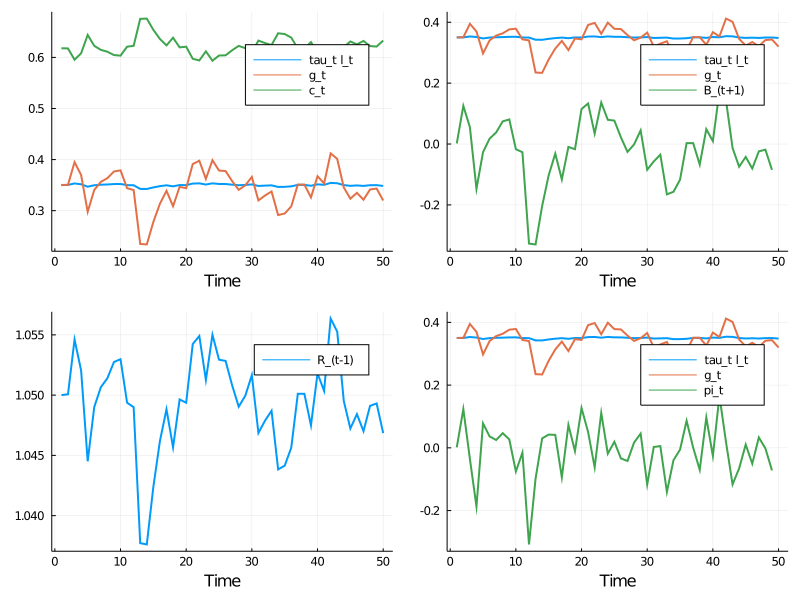The legends on the figures indicate the variables being tracked.

Most obvious from the figure is tax smoothing in the sense that tax revenue is much less variable than government expenditure

In :
gen_fig_2(path)

Out: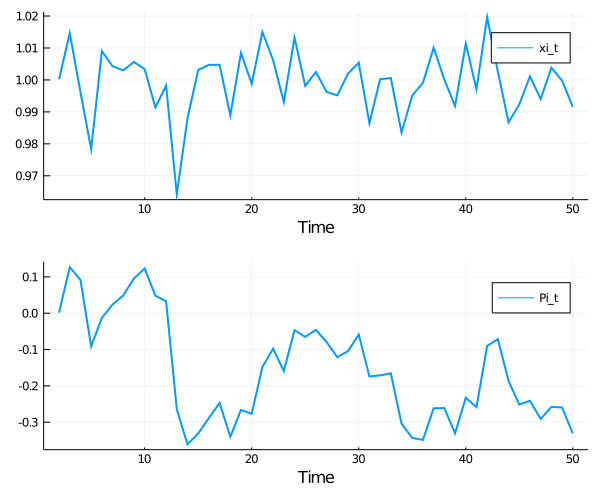See the original manuscript for comments and interpretation

### The Discrete Case¶

Our second example adopts a discrete Markov specification for the exogenous process

In :
# Parameters
β = 1 / 1.05
P = [0.8 0.2 0.0
0.0 0.5 0.5
0.0 0.0 1.0]

# Possible states of the world
# Each column is a state of the world. The rows are [g d b s 1]
x_vals = [0.5 0.5 0.25;
0.0 0.0  0.0;
2.2 2.2  2.2;
0.0 0.0  0.0;
1.0 1.0  1.0]
Sg = [1.0 0.0 0.0 0.0 0.0]
Sd = [0.0 1.0 0.0 0.0 0.0]
Sb = [0.0 0.0 1.0 0.0 0.0]
Ss = [0.0 0.0 0.0 1.0 0.0]
proc = DiscreteStochProcess(P, x_vals)

econ = Economy(β, Sg, Sd, Sb, Ss, proc)
T = 15
path = compute_paths(econ, T)

gen_fig_1(path)

Out: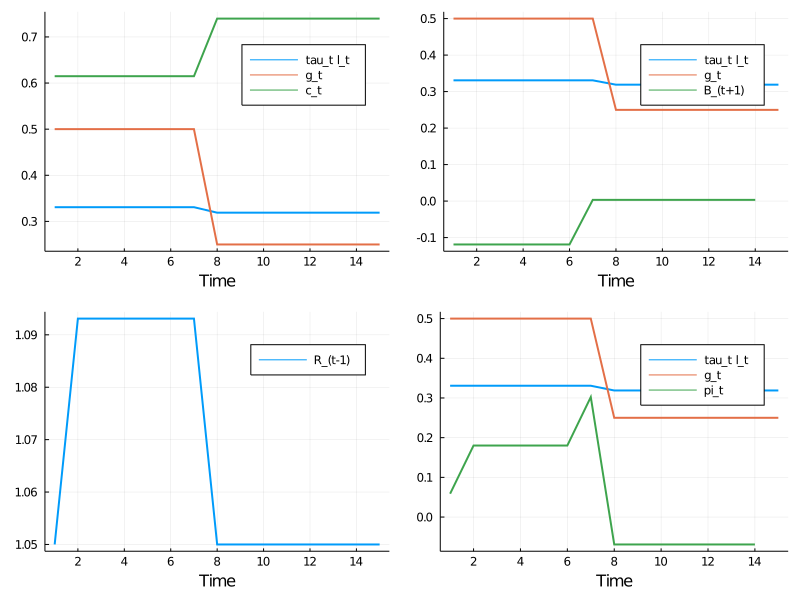The call gen_fig_2(path) generates

In :
gen_fig_2(path)

Out: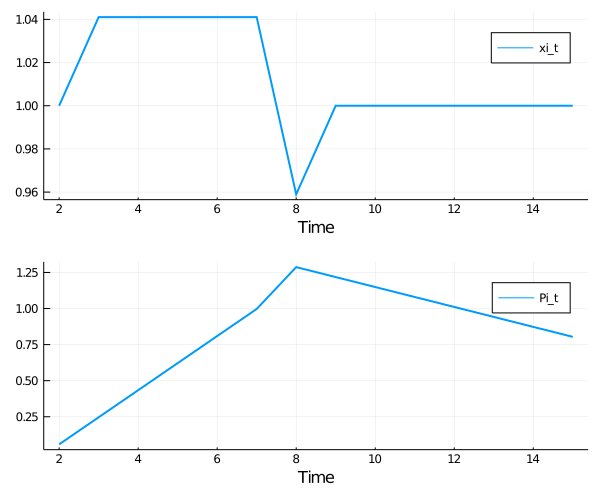See the original manuscript for comments and interpretation

## Exercises¶

### Exercise 1¶

Modify the VAR example given above, setting

$$g_{t+1} - \mu_g = \rho (g_{t-3} - \mu_g) + C_g w_{g, t+1}$$

with $\rho = 0.95$ and $C_g = 0.7 \sqrt{1 - \rho^2}$.

Produce the corresponding figures.

## Solutions¶

In :
# parameters
β = 1 / 1.05
ρ, mg = .95, .35
A = [0. 0. 0. ρ  mg*(1-ρ);
1. 0. 0. 0.       0.;
0. 1. 0. 0.       0.;
0. 0. 1. 0.       0.;
0. 0. 0. 0.       1.]
C = zeros(5, 5)
C[1, 1] = sqrt(1 - ρ^2) * mg / 8
Sg = [1. 0. 0. 0. 0.]
Sd = [0. 0. 0. 0. 0.]
Sb = [0. 0. 0. 0. 2.135]
Ss = [0. 0. 0. 0. 0.]
proc = ContStochProcess(A, C)
econ = Economy(β, Sg, Sd, Sb, Ss, proc)

T = 50
path = compute_paths(econ, T)

In :
gen_fig_1(path)

Out: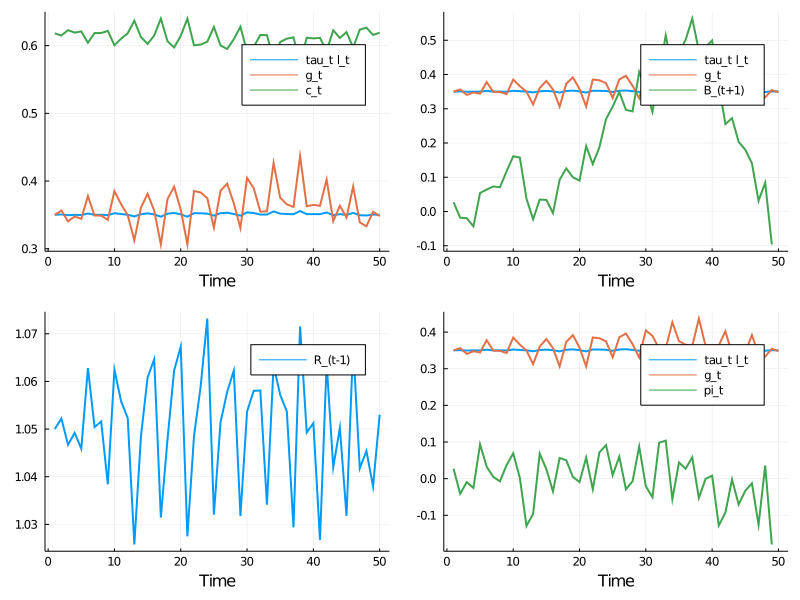In :
gen_fig_2(path)

Out: